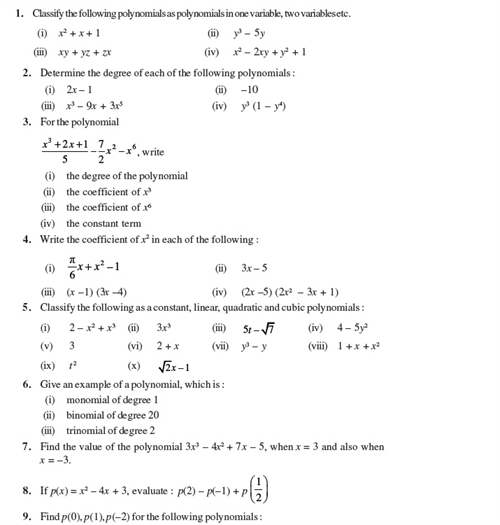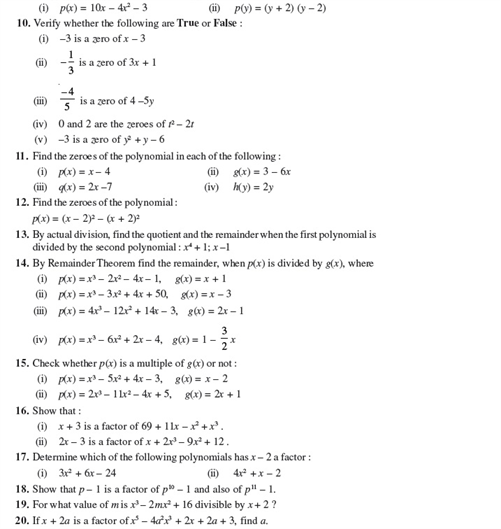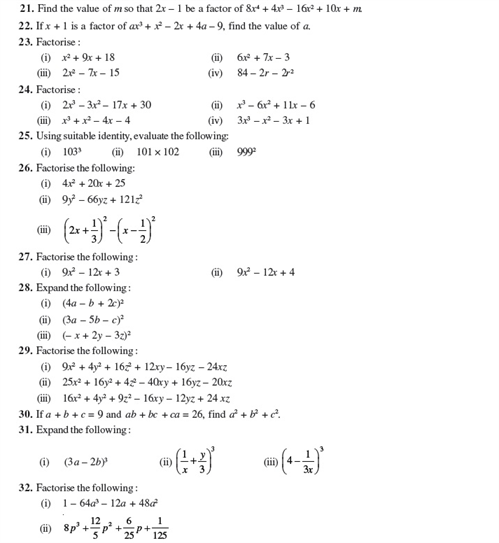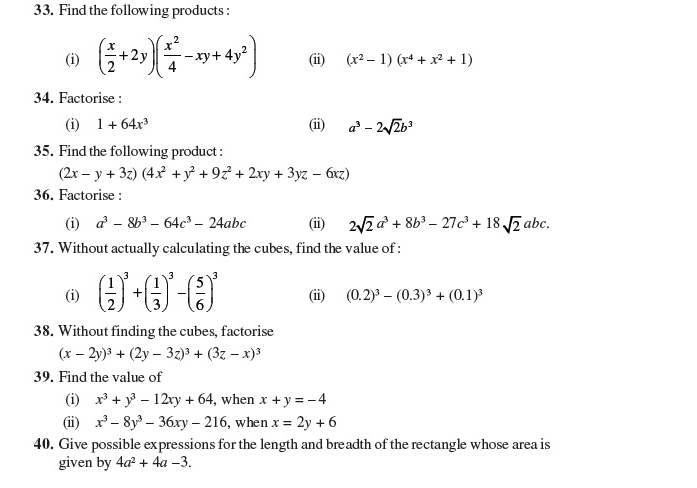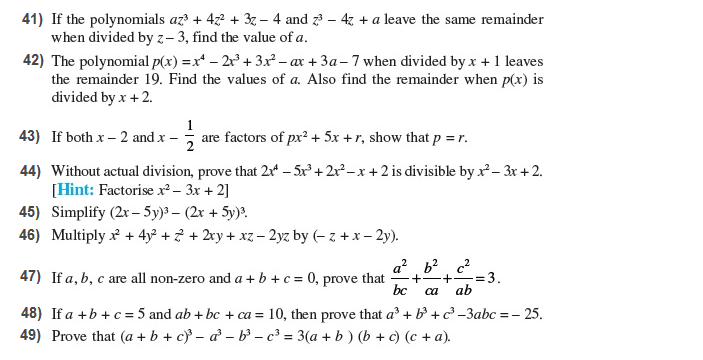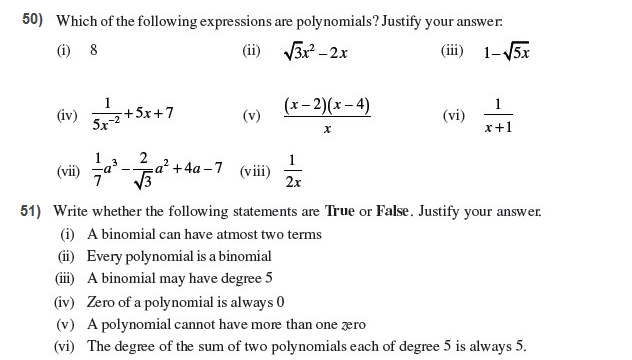# Important Questions Class 9 Maths Chapter 2 Polynomial

Important questions for Class 9 Maths Chapter 2 Polynomials are provided here to help the CBSE students score well in their class 9 maths exam. These practice questions from polynomials chapter (NCERT) will help the students to have a better understanding of the concepts and thus, develop their problem-solving skills.

Also Check:

## Important Polynomials Questions For Class 9- Chapter 2 (With Solutions)

Some important questions from polynomials are given below with solutions. These questions will help the 9th class students to get acquainted with a wide-variations of questions and develop the confidence to solve polynomial questions more efficiently.

1. Give an example of a monomial and a binomial having degrees as 82 and 99 respectively.

Solution:

An example of monomial having a degree of 82 = x82

An example of a binomial having a degree of 99 = x99 + x

2. Compute the value of 9x2 + 4y2 if xy = 6 and 3x + 2y = 12.

Solution:

Consider the equation 3x + 2y = 12

Now, square both sides:

(3x + 2y)2 = 122

=> 9x2 + 12xy + 4y2 = 144

=>9x2 + 4y2 = 144 – 12xy

From the questions, xy = 6

So,

9x2 + 4y2 = 144 – 72

Thus, the value of 9x2 + 4y2 = 72

6.   Find the value of the polynomial 5x – 4x2 + 3 at x = 2 and x = –1

Solution:

Let the equation be f(x) = 5x – 4x2 + 3

Now, for x = 2,

f(2) = 5(2) – 4(2)2 + 3

=> f(2) = 10 – 16 + 3 = –3

Or, the value of the polynomial 5x – 4x2 + 3 at x = 2 is -3

Similarly, for x = –1,

f(–1) = 5(–1) – 4(–1)2 + 3

=> f(–1) = –5 –4 + 3 = -6

4. Calculate the perimeter of a rectangle whose area is 25x2 – 35x + 12.

Solution:

Given,

Area of rectangle = 25x2 – 35x + 12

We know, area of rectangle = length × breadth

So, by factoring 25x2 – 35x + 12, the length and breadth can be obtained.

25x2 – 35x + 12 = 25x– 15x – 20x + 12

=> 25x2 – 35x + 12 = 5x(5x – 3) – 4(5x – 3)

=> 25x2 – 35x + 12 = (5x – 3)(5x – 4)

So, the length and breadth are (5x – 3)(5x – 4).

Now, perimeter = 2(length + breadth)

So, perimeter of the rectangle = 2((5x – 3)+(5x – 4))

= 5x – 3 + 5x – 4 = 10x – 7

So, the perimeter = 10x – 7

5. Find the value of x3 + y3 + z3 – 3xyz if x2 + y2 + z2 = 83 and x + y + z = 15

Solution:

Consider the equation x + y + z = 15

From algebraic identities, we know that (a + b + c)3 = a2 + b2 + c+ 2(ab + bc + ca)

So,

(x + y + z)3 = x+ y2 + z2 + 2(xy + yz + xz)

From the question, x2 + y2 + z2 = 83 and x + y + z = 15

So,

153 = 83 + 2(xy + yz + xz)

=> 225 – 83 = 2(xy + yz + xz)

Or, xy + yz + xz = 142/2 = 71

Using algebraic identity a³ + b³ + c³ – 3abc = (a + b + c)(a² + b² + c² – ab – bc – ca),

x3 + y3 + z3 – 3xyz = (x + y + z)(x² + y² + z² – (xy + yz + xz))

Now,

x + y + z = 15, x² + y² + z² = 83 and xy + yz + xz = 71

So, x3 + y3 + z3 – 3xyz = 15(83 – 71)

=> x3 + y3 + z3 – 3xyz = 15 × 12

Or, x3 + y3 + z3 – 3xyz = 180

### Extra Questions For Class 9 Chapter 2: Polynomials (NCERT)